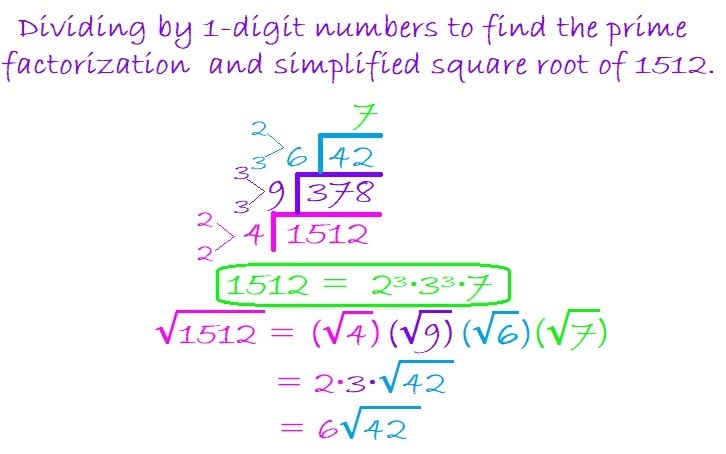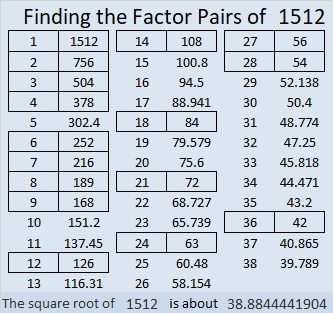# 1512 Bigger Bites of Cake

Contents

### Using the Cake Method:

I like using the cake method to find the prime factorization of a number. I also use it to find square roots.

If you know the multiplication table well, dividing by any number from 2 to 9 is not difficult.

It is easy to check to see if a number is divisible by 4 or by 9. And it is actually easier to divide by 4 once than it is to divide by 2 twice or to divide by 9 once than it is to divide by 3 twice. That way we get to take bigger bites of cake!

I know that 1512 is divisible by 4 because the number formed from the last two digits in order, 12, is divisible by 4.

I also know that 1512 is divisible by 9 because 1 + 5 + 1 + 2 = 9.

Thus, I’ll begin by dividing first by 4 and then the result by 9 as illustrated below:When my new divisor becomes 42, if I didn’t remember where it appears in the multiplication table, I would still be fine. I know that 42 is divisible by 2 because it is even and 3 because 4 + 2 = 6, a number divisible by 3.

Thus I can divide 42 by 6 because 2 × 3 = 6, and it is far easier to divide 42 by 6 than it is to divide it first by 2 and then by 3.

To take the square root of 1512, I simply take the square root of the numbers on the outside of the cake. 4 and 9 are perfect squares so I use their square roots. Neither 6 nor 7 has any perfect squares, so I just multiply them together to get 42.

Dividing 1512 this way allowed me to make just a three-layer cake instead of a 6-layer cake to find its prime factorization and its square root!

Some people prefer to do yard work more than having cake, so here is one of the MANY possible factor trees for 1512. Make sure you pick up all seven of the leaves with prime numbers wherever they are.### Factors of 1512:

• 1512 is a composite number.
• Prime factorization: 1512 = 2 × 2 × 2 × 3 × 3 × 3 × 7, which can be written 1512 = 2³ × 3³ × 7.
• 1512 has at least one exponent greater than 1 in its prime factorization so √1512 can be simplified. Taking the factor pair from the factor pair table below with the largest square number factor, we get √1512 = (√36)(√42) = 6√42.
• The exponents in the prime factorization are 2, 1, and 2. Adding one to each exponent and multiplying we get (3 + 1)(3 + 1)(1 + 1) = 4 × 4 × 2 = 32. Therefore 1512 has exactly 32 factors.
• The factors of 1512 are outlined with their factor pair partners in the graphic below.This site uses Akismet to reduce spam. Learn how your comment data is processed.